Refer to our Texas Go Math Grade 2 Answer Key Pdf to score good marks in the exams. Test yourself by practicing the problems from Texas Go Math Grade 2 Lesson 17.2 Answer Key Add and Subtract Lengths.

Essential Question
How can drawing a diagram help when solving problems about lengths?
Drawing diagrams is a useful problem solving heuristic. Diagrammatic representations have been shown to be superior to verbal representations when solving problems

Unlock the Problem
Nate had 23 centimeters of string. He gave 9 centimeters of string to Myra. How much string does Nate have now?

What information am I given?
Nate had __ centimeters of string.
He gave ______ centimeters of string to Myra.

Plan
What is my plan or strategy?
I can _______________ to show the problem.

Solve
Show how you solve the problem.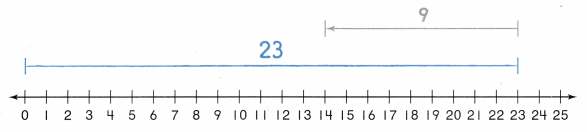Nate has ___ centimeters of string now.
Explanation:

What information am I given?
Nate had 23 centimeters of string.
He gave 9 centimeters of string to Myra.

Plan
What is my plan or strategy?
I can Draw to show the problem.

Solve
Show how you solve the problem.Nate has 14 centimeters of string now.
23 – 9 = 14

Home Connection

• Your child drew a diagram to represent a problem about lengths. The diagram can be used to choose the operation for solving the problem.

Draw a diagram. Then solve.

Question 1.
Ellie has a ribbon that is 12 centimeters long. Gwen has a ribbon that is 9 centimeters long. How many centimeters of ribbon do they have?

• What information am I given?
• What is my plan or strategy?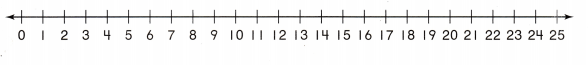They have ___ centimeters of ribbon.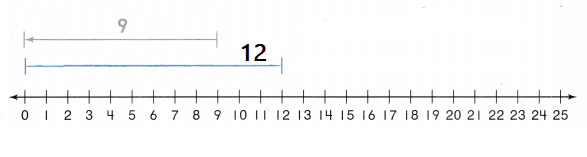Explanation:
They have 3 centimeters of ribbon.
total 12 centimeters
Gwen has a ribbon that is 9 centimeters long
12 – 9 = 3

Question 2.
A strip of paper is 24 centimeters long. Justin tears 8 centimeters off of the strip. How long is the strip of paper now?Now the strip of paper is ___ centimeters long.Explanation:
Total strip of paper is 24 centimeters long
24 – 8 = 16
16 centimeters long is the strip of paper now

Math Talk
Mathematical Processes
Explanation:
Ellie has a ribbon that is 12 centimeters long.
Gwen has a ribbon that is 9 centimeters long.
12  – 9 = 3
3 centimeters of ribbon  they have

Share and Show

Draw a diagram. Then solve.

Question 3.
A chain of paper clips is 18 centimeters long. Sondra adds 6 centimeters of paper clips to the chain. How long is the chain now?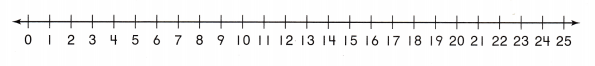The chain is ___ centimeters long now.Explanation:
A chain of paper clips is 18 centimeters long.
Sondra adds 6 centimeters of paper clips to the chain.
18 – 6 = 12
The chain is 12  centimeters long now.

Problem Solving

Question 4.
H.O.T. Multi-Step A chalk mark was 22 centimeters long. Greg erased part of the mark. Now it is 5 centimeters long. How many centimeters of the mark did Greg erase?Greg erased __ centimeters of the mark.
Greg erased 17 centimeters of the mark.Explanation:
A chalk mark was 22 centimeters long.
Greg erased part of the mark.
Now it is 5 centimeters long.
22 – 5 = 17 centimeters long.

Question 5.
H.O.T. Start at the point on the number line. Draw a diagram to stand for a length of 12 centimeters.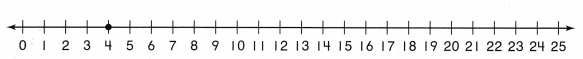Explanation:
Started from the point
The point started at 4
to draw the standard length of 12 centimeters.
16 – 12 = 4

Draw a diagram to solve.

Question 6.
Use Diagrams Ella has a piece of yarn that is 25 centimeters long. She uses
8 centimeters of it for a project. How many centimeters of yarn does she have now?Ella has ___ centimeters of yarn now.

• What information am I given?
• What is my plan or strategy?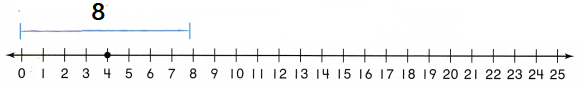Explanation:
Ella has a piece of yarn that is 25 centimeters long.
8 centimeters of it for a project.
25 – 8 = 17 centimeters long.
Ella has17 centimeters of yarn now.

Question 7.
Texas Test Prep Amber has a blue ribbon that she measures in centimeters. She draws this diagram to show the length of the ribbon. How many centimeters long is her ribbon?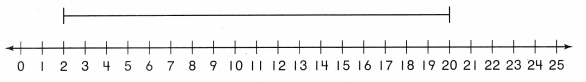(A) 20 centimeters
(B) 18 centimeters
(C) 22 centimeters
Explanation:
20 – 2 = 18 centimeters

Take Home Activity

• Have your child explain how he or she used a diagram to solve a problem in this lesson.
Explanation:
By measuring with the centimeter number line.

### Texas Go Math Grade 2 Lesson 17.2 Homework and Practice Answer Key

Draw a diagram. Then solve.

Question 1.
Mary Beth has a ribbon that is 15 centimeters long. Alicia has a ribbon that is 9 centimeters long. How many centimeters of ribbon do they have?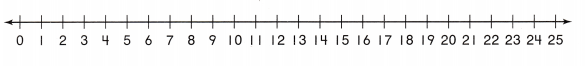They have ___ centimeters of ribbon.They have 24 centimeters of ribbon.
Explanation:
Mary Beth has a ribbon that is 15 centimeters long.
Alicia has a ribbon that is 9 centimeters long.
15 + 9 = 24

Problem Solving

Question 2.
Multi-Step A string is 21 centimeters long. Sue cuts off a piece of the string. Now the string is only 8 centimeters long. How many centimeters of string did Sue cut off?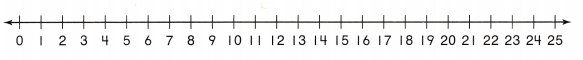Sue cut off ___ centimeters of string.
Sue cut off 13 centimeters of string.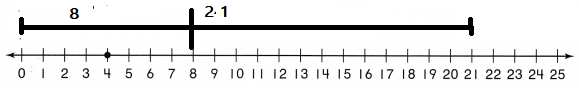Explanation:
A string is 21 centimeters long.
Sue cuts off a piece of the string.
Now the string is only 8 centimeters long.
21 – 8 = 13

Texas Test Prep

Lesson Check

Question 3.
Faith has a ribbon that she measures in centimeters. She draws this diagram to show the length of the ribbon. How many centimeters long is her ribbon?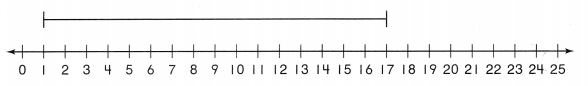(A) 19 centimeters
(B) 16 centimeters
(A) 17 centimeters
Explanation:
17 – 1 = 16 centimeters
16 centimeters long is her ribbon

Question 4.
Jim draws a diagram to show the length of his two chains. How many centimeters of chain does Jim have?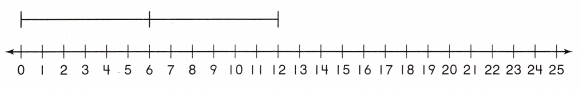(A) 12 centimeters
(B) 8 centimeters
(C) 10 centimeters
Explanation :
6 + 6 = 12
Jim have 12 centimeters of chain

Question 5.
Multi-Step Jan has a piece of yarn that is 24 centimeters long. She uses 6 centimeters of it for a project and 9 centimeters of it for another project. How many centimeters of yarn does she have left?(A) 9 centimeters
(B) 19 centimeters
(C) 15 centimeters